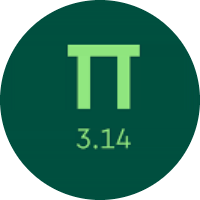# Learning The Two Dimensional Figures

In this quiz, you will learn about two-dimensional figures. You will learn about regular and irregular polygons, parallel, perpendicular, intersecting lines, types of angles, and the properties of polygons.

Start Quiz

What kind of triangle is this?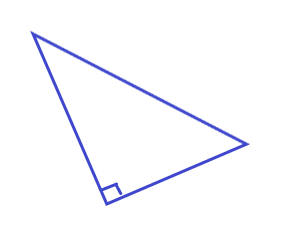acute

obtuse

right

Estimate the measure of this angle.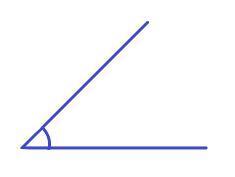45

90

120

Can the sides of a triangle have lengths 1, 1, and 9?

yes

no

Choose the word that makes this sentence true.

A rhombus is ##sometimes## a rectangle.

sometimes never always

What is the measure of the missing angle?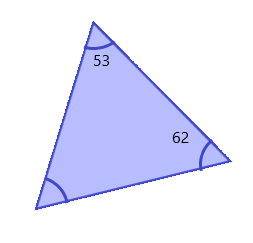65

55

58

Is this shape a regular polygon?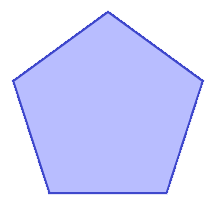yes

no

A right triangle has one angle that measures 20°. What is the measure of the other acute angle?

60

50

70

What is the measure of the missing angle?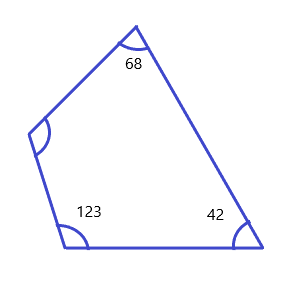124॰

127॰

132॰

Name this angle.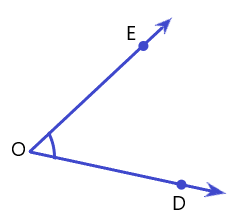EOD

ODE

The measure of an angle is 57°. What is the measure of its complementary angle?

33॰

43॰

45॰

What is the value of q?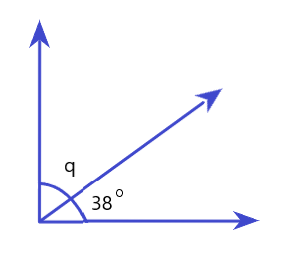32॰

45॰

52॰

Quiz/Test Summary
Title: Learning The Two Dimensional Figures
Questions: 11
Contributed by: